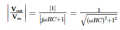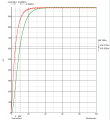# 2nd Order passive Low Pass Filter

#### Alexandru Chiser

Joined Feb 16, 2017
44
The 2nd filter acts as a LOAD for the first filter. So, this load has an impedance equal to R2 + 1/jwC. Basically, at the first filters output we connect an impedance equal to R2 + 1/jwc. Right?

#### AnalogKid

Joined Aug 1, 2013
8,111
Right.

AND, this is why if you do things this way the 2nd stage impedance should be at least 10x the first stage impedance. The higher the impedance of the 2nd stage, the less it loads and alters the corner frequency of the first stage. 10x is a standard engineering *approximation* for the beginning of the range where things do not matter. When one impedance is in parallel with another, the loading effect is officially "relatively small" at a ratio of 10x. Obviously, 50x or 100x would be even less loading. And, how much loading is too much depends on the actual circuit and its required performance.

ak

#### Alexandru Chiser

Joined Feb 16, 2017
44
Okay, but now i am using an op-amp as a buffer to isolate the 2 filters, so i dont need to increase the impedance of the 2nd stage 10x.

#### crutschow

Joined Mar 14, 2008
23,382
Okay, but now i am using an op-amp as a buffer to isolate the 2 filters, so i dont need to increase the impedance of the 2nd stage 10x.
Correct.

#### Bordodynov

Joined May 20, 2015
2,402

#### Alexandru Chiser

Joined Feb 16, 2017
44
Ive done some tests with the following configurations:

1) 2 1st order filters cascaded

Calculus: 33Hz - Simulation: 14Hz - Reality: 14Hz

2) 2 1st order filters isolated by op-amp

Calculus: 33Hz - simulation: 22Hz - Reality: 23Hz

So the 2nd version is better because is closer to the ideal case

Bordodynov: i simulated the same circuits, but with different values. I obtained the same results (ofc, different frequency, but the characteristics were the same).#### crutschow

Joined Mar 14, 2008
23,382
Calculus: 33Hz - simulation: 22Hz - Reality: 23Hz
You calculus is off.
Remember that the standard 1/2piRC calculation is the -3db point of one RC filter.
For two filters in series that gives the -6dB point, with the -3dB point at about 22Hz as the simulation and measurements show.
So to get the -3dB point of the combined filter, you must calculate the -1.5dB point of each filter.

#### Alexandru Chiser

Joined Feb 16, 2017
44
You calculus is off.
Remember that the standard 1/2piRC calculation is the -3db point of one RC filter.
For two filters in series that gives the -6dB point, with the -3dB point at about 22Hz as the simulation and measurements show.
So to get the -3dB point of the combined filter, you must calculate the -1.5dB point of each filter.
And how do i do that? Whats the formula?

PS: in the simulation the -6dB point is exactly at 33Hz...

PS2: so.. for a 2nd order filter, i should look for the -6dB or the -3dB to find the cut-off frequency?

PS3: in the simulation the phase of the first filter at -3dB is -45*, and for both filters at -6dB is -90*...

Last edited:

#### crutschow

Joined Mar 14, 2008
23,382
And how do i do that? Whats the formula?
The -3dB point for the combined filter is where each filter has a rolloff of -1.5dB or 0.8414.
The transfer function of an RC low-pass filter isso you solve the equation for the frequency where Vout/Vin = 0.8444.
I'll leave that algebraic manipulation as a exercise for the reader.PS2: so.. for a 2nd order filter, i should look for the -6dB or the -3dB to find the cut-off frequency?
Normally the cutoff frequency is defined as the -3dB point.
PS3: in the simulation the phase of the first filter at -3dB is -45*, and for both filters at -6dB is -90*...

What's to explain?
The phase-shift of a single-pole RC filer is 45° at the -3dB point.
The phase-shifts of the two filters in series add together at any frequency.

#### Alexandru Chiser

Joined Feb 16, 2017
44
So... ive done the algebra and i came up to this:

0.841395 = 1 / sqrt(1 + w^2R^2C^2)
0.841395^2 = 1^2 / 1 + w^2R^2C^2
1 + w^2R^2C^2 = 1 / 0.841395^2

=> w^2R^2C^2 = 0.412 => w = sqrt(0.412 / R^2C^2) => w = 0.64 / 10K * 470nf = 136.63

w = 2piF => F = 21.74HZ.

This is indeed the frequency at -1.5dB FOR ONE FILTER, as the simulation shows

But for 2 filters, the transfer function is:

1 / (1+jwRC) * 1 / (1+jwRC) (you multiply the transfer functions of the both filters), this being equal to: 1 / (1 +2jwRC - w^2R^2C^2) and... im stuck here... I dont know if its okay and i should calculate for -3dB (Vout/Vin = 0.707). Im stuck at calculating the absolute value of the denominator...

#### crutschow

Joined Mar 14, 2008
23,382
I dont know if its okay and i should calculate for -3dB (Vout/Vin = 0.707). Im stuck at calculating the absolute value of the denominator..
Well, I'm rather allergic to doing mathso you're on you own here, or perhaps someone else will help.

#### Alexandru Chiser

Joined Feb 16, 2017
44
Regarding the time constant,

A 1st order filter has T = RC. In my case, both of the filters have the same time constant. But whats the time constant of the whole system? Im pretty sure its a little bit bigger than the time constant of 1 filter, maybe 2 times?

Theory says: time constant is where the Capacitor charges at 63%. So i simulated my circuit, where i apply a 1V amplitude PWM signal, with 98% duty cycle. This means that the voltage at the output of the filter is 0.98V. 63% means = 0.6174. This is the simulation:RED line - 1st order filter (the first filter, before the op-amp) - it has a time constant of aprox 4.67ms
GREEN line - the output of the whole circuit - it has a time constant of aprox 10.02ms...

#### crutschow

Joined Mar 14, 2008
23,382
From Wikipedia:
In physics and engineering, the time constant, usually denoted by the Greek letter τ (tau), is the parameter characterizing the response to a step input of a first-order, linear time-invariant (LTI) system.
Thus the time-constant only refers to the response of a first-order circuit with one RC (or RL).
It does not apply to your circuit with two cascaded RC filters.

#### Alexandru Chiser

Joined Feb 16, 2017
44
[QUOTE="crutschow, post: 1250133, member: 19451"
It does not apply to your circuit with two cascaded RC filters.[/QUOTE]

But, as you can see... the voltage stabilizes at different times (depending where i measure, at the output of the first, or the second filter). I know that the ripple is lower, but why does it take longer to stabilize at the output of the whole system?

#### crutschow

Joined Mar 14, 2008
23,382
why does it take longer to stabilize at the output of the whole system?
Because you are adding together the settling time of two time-constants in series.
The more you add in series, the longer it will take to settle.